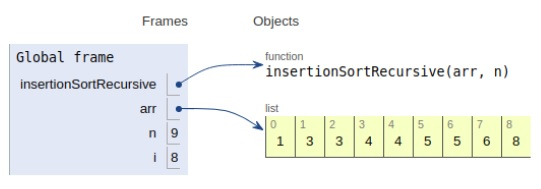# Python Program for Recursive Insertion Sort

Problem statement− We are given an array, we need to sort it using the concept of recursive insertion sort.

Insertion sort works on creating a parallel array in which we manually insert the elements in the specified order.

Now let’s observe the solution in the implementation below −

## Example

Live Demo

# recursive way
def insertionSortRecursive(arr,n):
# base case
if n<=1:
return
# Sort
insertionSortRecursive(arr,n-1)
last = arr[n-1]
j = n-2
while (j>=0 and arr[j]>last):
arr[j+1] = arr[j]
j = j-1
arr[j+1]=last
# main
arr = [1,5,3,4,8,6,3,4,5]
n = len(arr)
insertionSortRecursive(arr, n)
print("Sorted array is:")
for i in range(n):
print(arr[i],end=" ")

## Output

Sorted array is :
1 3 3 4 4 5 5 6 8All the variables are declared in the local scope and their references are seen in the figure above.

## Conclusion

In this article, we have learned about how we can make a Python Program for Recursive Insertion Sort